## Complex IFStarted by 1 year ago20 replieslatest reply 1 year ago184 views

Hello

I am building up a RF transceiver using integrated up/down converter chip.

This particular chip requires a complex Intermediate Frequency as inputs for up conversion (Tx-IF-I, Tx-IF-Q).  As such an external 3dB splitter/hybrid combiner e.g. Mini Circuit QCH-63+ is used to convert real IF to complex IF (I+jQ).

Due to the physical implementation, the

0-degree pin of the combiner is facing the Tx-IF-Q (up converter)

and the 90-degree pin/combiner is facing Tx-IF-I (up converter).

To avoid cross-over, is it ok to connect as they are. Will the upconverted waveform shift?

0-degree/combiner > Tx-IF-Q (up converter)

90-Degree/combiner > Tx-IF-I (up converter)

Also, how do I evaluate EVM due to gain/phase imbalances due to combiner and imperfection in the modulator.

I am just curious why the modulator cannot just accept real IF and for the complex conversion inside the chip.

[ - ]The purpose I & Q is to keep track of phase.  If you swap real and imaginary you just twist the phase by 90 degrees.  The amplitude is still the same.  The measurements you get out will still be the same, you have just "renamed" real to be imaginary and vice versa.  Since phase is Atan(imgaginary/real) you have the inverse - the Atan(real/imaginary) = 1/Atan(imaginary/real).   If you really care about the input phase of the signal, you can still find what it is.

As for why they use complex input and output, it's easier for them for one thing, and for another they don't know what is important to you as the user for the conversion to the IF.  Too many variables they don't want to deal with.

Hook it up and play - If things look upside down it's because they are!

[ - ]Hello

I done some calculations just to prove for myself but the angles calculated are different.

Real=0.5. Imag=0.9

Phase = arctan (0.9/0.5) = 60.9deg

now if I inverse due to the physical implementation

Phase= arctan (0.5/0.9) = 29.05deg.

. I.e. the angles haven’t twisted by 90 deg. Also, how do I assess EVM due to gain and phase imbalances. Thank you
[ - ]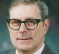Your angles 60.9 deg and 29.05 deg add up to 90 deg within a probably rounding error. The phase has not been "twisted" but reflected.

[ - ]Exactly!  $atan(x)=\pi - atan(1/x)$  So the term "twisted" implies a positive rather than negative shift.  "Reflected" is a better term.

The gain in the EVM should be the same.  The phase imbalances will be reflected by 90 degrees.  Since you have a "real" signal for input, the phase doesn't matter so much.  However, if there is a phase shift that is different in each arm (I or Q) then you will get different results from swapping the inputs.  If you are operating in the GHz range it would be hard to do the experiment of swapping the inputs to check.  If you can, do that experiment of swapping the I and Q so you can see what happens and understand the math behind it.  It won't be exactly the same, but it should be close.  Unless there's a problem, and that tells you something too.

[ - ]$atan(x)=\pi/2 - atan(1/x)$
[ - ]The real signal can be thought of as if there are 2 complex signals one with a constellation point rotating in a positive counter-clock wise rotation and one with a negative clock wise rotation, both at the same rads/sec but in equal and opposite directions so they cancel the imaginary part out. selecting the IQ pairs that go into the up-converter will select one of these signals. I think of it as one being the upper side band and the other the lower side band, with respect to the carrier. You can simulate this in matlab or python by just encoding a tone then up-converting it. Either by multiplying your complex signal by -i,i or through hilbert transform you can rotate the signal to see some of the affects.

[ - ]Many thanks for the explanation.

Do I take it the overall waveform would be the same on the spectrum analyser (upconverted signal at f1, image)?

For Rx down-conversion, I have to convert the complex IF back to real IF.

Do I ensure that I (ftom down-conversion is fed to Q of combiner and Q (from downconversion) to I?

Can someone point to how I implement this on Matlab or Python?

Thank you

[ - ]This matlab code can help modelling your thoughts:

clc;clear;

x = randn(1,2^10);

x = hilbert(x);

fup = exp(j*2*pi*(0:length(x)-1)*.3);

xup = x.*fup;

fdn = exp(j*2*pi*(0:length(x)-1)*-.3);

xdn = xup.*fdn;

plot(real(x));hold;

plot(real(xdn),'g--');

%

[ - ]Post deleted by author

[ - ]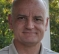Many excellent answers here, but 1 item I think has not been mentioned.
The word reflection is used in several of them, and that is the essence of my comment.
An exchange of I and Q is in fact a CONJUGATE with a 90 degree phase rotation.

As mentioned, you probably don't care about the absolute phase, so the 90 degree rotation probably does not matter.

However, it is not clear to me whether that conjugate makes a difference for you.

[ - ]I read one of the message that swapping I and Q (like in the case described earlier)effectively spectrally invert the waveform.

Swapping I and Q reverses all the frequencies. For example, a signal 5 kHz above the mixer's LO will appear at -5 kHz, instead of 5kHz

My concern would I be able to see the up converted signal on the spectrum analyser as the waveform us spectrally inverted in negative domain.

[ - ]I'm not sure that I can accurately visualize the issue you are facing, but conjugation is exactly the same as rotating in reverse.

exp(-1j*2pi*fc) vs. exp(+1j*2pi*fc)

[ - ]Please excuse the long msg but a picture paints a 1000 words (really 1024 to be exact!) I'm including code below not sure now to make it show up in a separate window. In the code I have created a msg it is nothing more than a 1MHz sine wave. lets call it the IF signal. If we take the real part and perform a PSD on it it looks like thisIf we take a hilbert transform of the msg it and display ittaking the real parts of the carrier and msg mixing them you get this:

Note the positive and negative images for both the carrier and the up-converted msg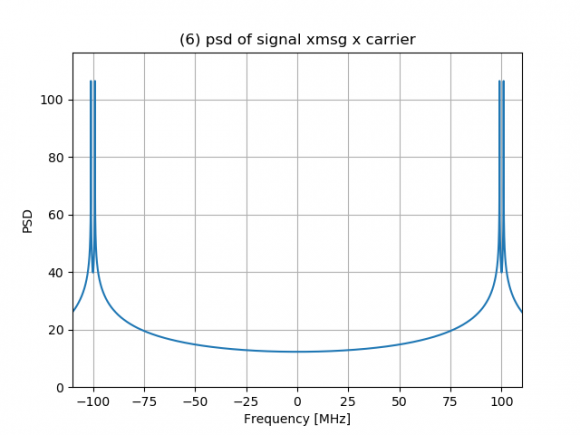OK now for the punch line, here is the complex upconvert.101MHz as expected. So taking the real and imaginary parts and swapping them produces this: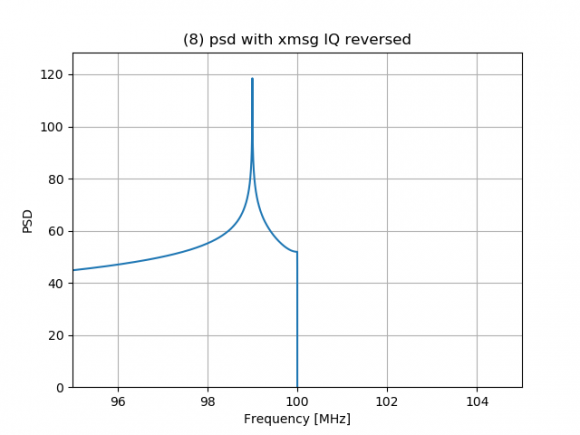Not sure if this helps but nice to look at some diagrams. Here is the code in python if you want to reproduce it.

import numpy as np
import matplotlib.pyplot as plt
from scipy.signal import hilbert

def plot_data(data, names, points=False):
"""
function plots n rows of data based on list length

data : list of data to plot
names : list of names of data
title : plot name and file name.
"""
if len(data) != len(names):
print('list of data and names are not equal length')
return
else:
fig, ax = plt.subplots(len(data), 1)  # N by 1 subplots

title = ' '.join(names)
# plt.title(title)

for i in range(len(data)):
ax[i].plot(data[i])
if points:
ax[i].plot(data[i], 'r.')
ax[i].grid('on')
# ax[i].legend()
ax[i].set_ylabel(names[i])

plt.show(block=False)
plt.savefig(title+'.png')

def plot_iq_data2x(data1, data2, name1, name2):
"""
function plots 2 rows of data, 1 for real, and 1 for imaginary
data1,2 : complex data to plot
"""
fig, (ax1, ax2) = plt.subplots(2, 1)
ax1.plot(np.real(data1), 'b', label='real')
ax1.plot(np.real(data1), 'r.')
ax1.plot(np.imag(data1), 'g', label='imag')
ax1.plot(np.imag(data1), 'r.')
ax1.grid('on')
ax1.legend()
ax2.plot(np.real(data2), 'b', label='real')
ax2.plot(np.real(data2), 'r.')
ax2.plot(np.imag(data2), 'g', label='imag')
ax2.plot(np.imag(data2), 'r.')
ax2.grid('on')
ax2.legend()
ax1.set_ylabel(name1)
ax2.set_ylabel(name2)
# ax1.set_xlim([-Tb, 4 * Tb])
# ax2.set_xlim([-Tb, 4 * Tb])
plt.legend()
plt.show(block=False)
plt.savefig(name1+'_'+name2+'.png')

def plot_psd(samples, Fs, plt_title, xlim=None):
# Calculate power spectral density (frequency domain version of signal)
psd = np.abs(np.fft.fftshift(np.fft.fft(samples))) ** 2
psd_dB = 10 * np.log10(psd)
freqs = np.linspace(Fs/-2, Fs/2, len(psd))

# Plot freq domain
plt.figure()
plt.plot(freqs, psd_dB)
plt.xlabel("Frequency [MHz]")
plt.ylabel("PSD")
if xlim:
plt.xlim(xlim)
plt.ylim([0, np.max(psd_dB)+10])
plt.grid('on')
plt.title(plt_title)
plt.show(block=False)
plt.savefig(plt_title+'.png')

def plot_iq_psd(x, fs, name):
zoom_len = int(len(x)/10)
plot_iq_data2x(x, x[0:zoom_len], name, 'zoom')
psd_name = name + '_' +str(fs)+'_sample_rate'
plot_psd(x, fs, psd_name)
plt.savefig(name+'.png')

# ---- start here --------
if __name__ == '__main__':
# make your over sample rate based on how many times a cycle you want a data point for viewing.
# note all numbers assume to be in MHz
Fc = 100  # carrier freq set to 100MHz
N = 2**16  # number of IF cycles to simulate
os = 16   # number of samples per cycle
Fs = os * Fc
Ts = 1 / Fs

# generate your time base starting at time zero
t = np.arange(0, os*N) / Fs

# generate the message, for here you are just using a tone at Fmsg
Fmsg = 1  # generate a 1 MHz tone
xmsg = np.cos(2 * np.pi * Fmsg * t)
plot_psd(xmsg[0::100], Fs/100, '(1) psd of xmsg')

xmsg_cmplx = hilbert(xmsg)
plot_psd(xmsg_cmplx[0::100], Fs / 100, '(2) psd of complex xmsg')
plot_iq_psd(xmsg_cmplx[0:2**16:16], Fs/16, '(3) IQ of xmsg complex')

# take the real parts of the msg and carrier and mix
x0 = xmsg * np.sin(2 * np.pi * Fc * t)

plot_data([x0[0:2048], xmsg[0:2048]], ['(5) real part x0', '(5)real part xmsg'], True)
plot_psd(x0, Fs, '(6) psd of signal xmsg x carrier', xlim=[-110, 110])

# complex mix
x1 = xmsg_cmplx * (np.cos(2*np.pi*Fc*t) + 1j*np.sin(2*np.pi*Fc*t))
plot_psd(x1, Fs, '(7) psd of complex up convert', xlim=[95,105])

# now reverse IQ on the msg signal
xmsg_rev = np.imag(xmsg_cmplx) + 1j*np.real(xmsg_cmplx)
x2 = xmsg_rev * (np.cos(2*np.pi*Fc*t) + 1j*np.sin(2*np.pi*Fc*t))
plot_psd(x2, Fs, '(8) psd with xmsg IQ reversed', xlim=[95, 105])

print('end')
[ - ]Thank you for the code.

I like to  get a common understanding.

I can see in the last graph that the spectrum have been inverted due to I and Q being reversed so 101MHz being reflected to 99MHz as a result.

Why is it in Fig.6 that the spectrum is not 99MHz and +101MHz as a result of mixing 1MHz with 100MHz LO? Can you please clarify?

[ - ]In Figure 6 it is a visualization of the real parts of each signal, the xmsg and the carrier multiplied together. On the positive side of the spectrum you have carrier +/- the msg  so 99, 101 and on the negative side of the spectrum you have -99 and -101. If you run the code you can zoom into the figures. You may need to use a debugger and place a break point on the figure is plotted that you want to examine or change "block=False" to "block=True" on the figure you want the sim to stop on in the plt.show() statement.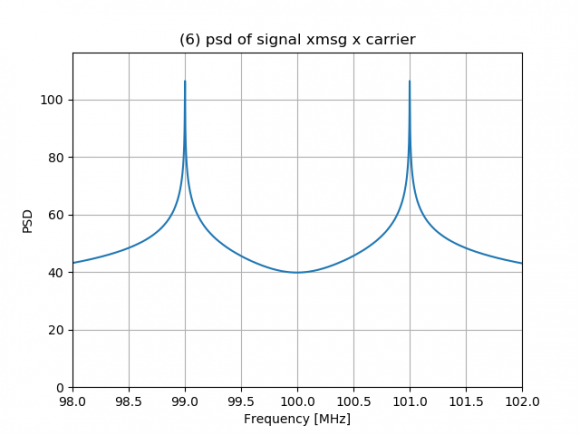[ - ]I get an error when I run the code in Spyder.

divide by zero encountered in log10 psd_dB = 10 * np.log10(psd)

[ - ]This is in reference to Python.

I also ran the Matlab code.  Does it just plot one graph (Fig.1) showing a complex waveform?

[ - ]I'm using pycharm. For some reason spyder will not run on my machine. Possibly check the version of numpy and update it. I have had issues with old versions of numpy.

In the IDE on the command line you could try just executing the commands you would need to make the array psd then pass it to the numpy function so numpy.log10()  after you import numpy.

[ - ]